• C语言整型转字符串 顺序存储顺序打印 #include <stdio.h> int main() { int num = 110086; char str = {0}; int divnum = 100000; int i = 0; for(i=0; i<6; i++) { str[i] = (num / divnum)+48...
C语言整型转字符串
顺序存储顺序打印
#include <stdio.h>

int main()
{
int num = 110086;
char str = {0};
int divnum = 100000;
int i = 0;
for(i=0; i<6; i++)
{
str[i] = (num / divnum)+48;
num = num % divnum;
divnum = divnum / 10;
}
return 0;
}

逆序存储顺序打印
#include <stdio.h>

int main()
{
int num = 110086;
char str = {0};
int i = 0;
for(i=5; i>=0; i--)
{
str[i] = (num % 10)+48;
num = num / 10;
}
for(i=0; i<6; i++)
{
printf("%s", str);
}
printf("\n");
return 0;
}

注意：整型变量与字符变量相加减是使用ASCII码值，可以通过类型转换或格式字符来控制打印。


展开全文• 这是我课余时间写的源码，暂时没有发现bug，如果您在使用过程中发现了bug，欢迎改正，同时欢迎您对此源码进行优化
• ## C语言 整数与字符串的相互转换

万次阅读 多人点赞 2018-06-26 23:22:57
C语言 整数与字符串的相互转换 ...二、整数转字符串  1、拓展函数itoa  itoa (表示 integer to alphanumeric)是把整型数转换成字符串的一个函数。  windows环境下，在&lt;stdlib.h&gt;头文件中有 c...
C语言 整数与字符串的相互转换

一、简述

C语言中整数与字符串的相互转换，有广泛应用的拓展函数(非标准库)，也可以自己尝试简单的实现。

二、整数转字符串

1、拓展函数itoa

itoa (表示 integer to alphanumeric)是把整型数转换成字符串的一个函数。

windows环境下，在<stdlib.h>头文件中有

char* itoa(int value,char*string,int radix);//value: 要转换的整数，string: 转换后的字符串,radix: 转换进制数，如2,8,10,16 进制等。

函数源码：

char* itoa(int num,char* str,int radix)
{
char index[]="0123456789ABCDEFGHIJKLMNOPQRSTUVWXYZ";//索引表
unsigned unum;//存放要转换的整数的绝对值,转换的整数可能是负数
int i=0,j,k;//i用来指示设置字符串相应位，转换之后i其实就是字符串的长度；转换后顺序是逆序的，有正负的情况，k用来指示调整顺序的开始位置;j用来指示调整顺序时的交换。

//获取要转换的整数的绝对值
{
unum=(unsigned)-num;//将num的绝对值赋给unum
str[i++]='-';//在字符串最前面设置为'-'号，并且索引加1
}
else unum=(unsigned)num;//若是num为正，直接赋值给unum

//转换部分，注意转换后是逆序的
do
{

}while(unum);//直至unum为0退出循环

str[i]='\0';//在字符串最后添加'\0'字符，c语言字符串以'\0'结束。

//将顺序调整过来
if(str=='-') k=1;//如果是负数，符号不用调整，从符号后面开始调整
else k=0;//不是负数，全部都要调整

char temp;//临时变量，交换两个值时用到
for(j=k;j<=(i-1)/2;j++)//头尾一一对称交换，i其实就是字符串的长度，索引最大值比长度少1
{
temp=str[j];//头部赋值给临时变量
str[j]=str[i-1+k-j];//尾部赋值给头部
str[i-1+k-j]=temp;//将临时变量的值(其实就是之前的头部值)赋给尾部
}

return str;//返回转换后的字符串
}

示例程序：

#include <stdlib.h>
#include <stdio.h>
int main()
{
int number1 = 123456;
int number2 = -123456;
char string = {0};
itoa(number1,string,10);
printf("数字：%d 转换后的字符串为：%s\n",number1,string);
itoa(number2,string,10);
printf("数字：%d 转换后的字符串为：%s\n",number2,string);
return 0;
}

效果截图：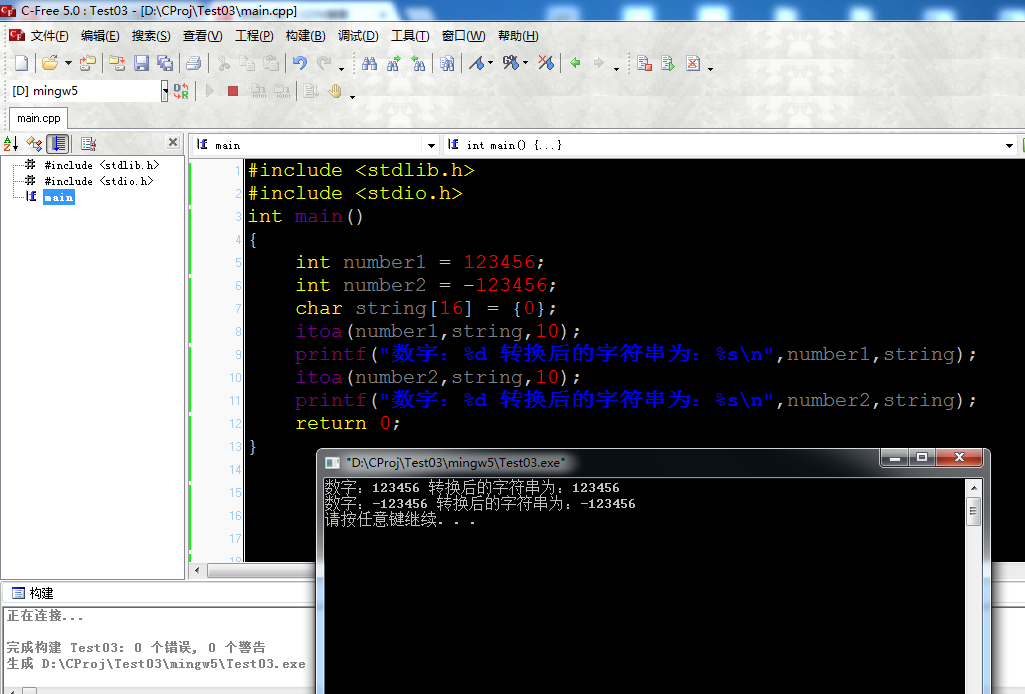2、自己简单实现

实现代码


#include <stdio.h>

char* Int2String(int num,char *str);//函数声明
int main()
{
int number1 = 123456;
int number2 = -123456;
char string = {0};
Int2String(number1,string);
printf("数字：%d 转换后的字符串为：%s\n",number1,string);
Int2String(number2,string);
printf("数字：%d 转换后的字符串为：%s\n",number2,string);
return 0;
}

char* Int2String(int num,char *str)//10进制
{
int i = 0;//指示填充str
if(num<0)//如果num为负数，将num变正
{
num = -num;
str[i++] = '-';
}
//转换
do
{
str[i++] = num%10+48;//取num最低位 字符0~9的ASCII码是48~57；简单来说数字0+48=48，ASCII码对应字符'0'
num /= 10;//去掉最低位
}while(num);//num不为0继续循环

str[i] = '\0';

//确定开始调整的位置
int j = 0;
if(str=='-')//如果有负号，负号不用调整
{
j = 1;//从第二位开始调整
++i;//由于有负号，所以交换的对称轴也要后移1位
}
//对称交换
for(;j<i/2;j++)
{
//对称交换两端的值 其实就是省下中间变量交换a+b的值：a=a+b;b=a-b;a=a-b;
str[j] = str[j] + str[i-1-j];
str[i-1-j] = str[j] - str[i-1-j];
str[j] = str[j] - str[i-1-j];
}

return str;//返回转换后的值
}

效果截图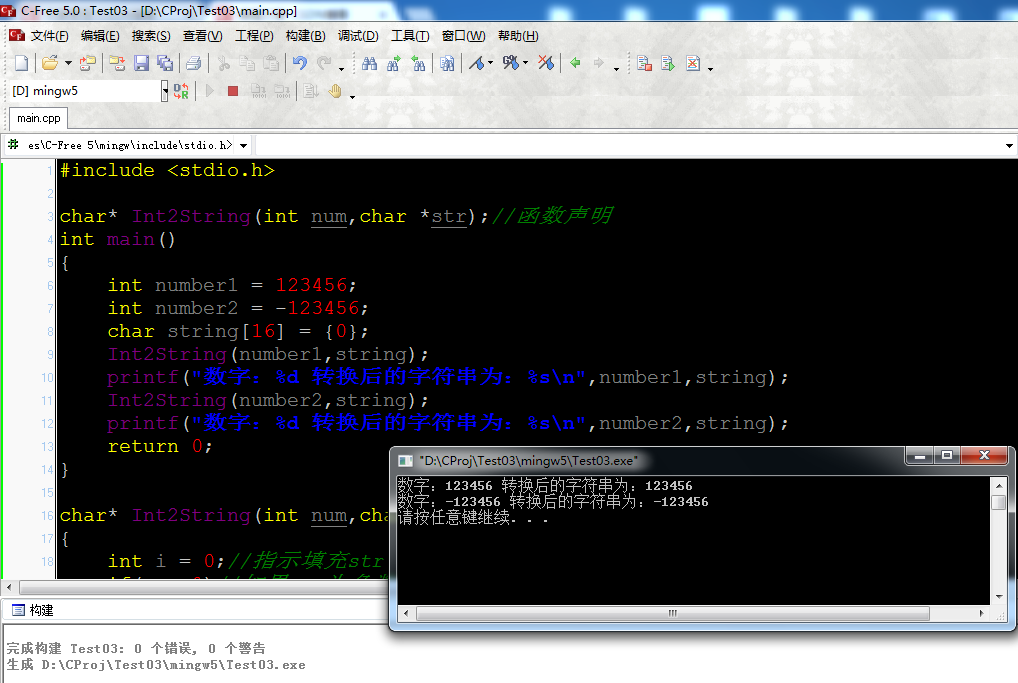三、字符串转整数

1、拓展函数
atoi (表示 alphanumeric to integer)是把字符串转换成整型数的一个函数。
windows环境下，在<stdlib.h>头文件中

int  atoi(const char *nptr);//字符串转整数函数，nptr: 要转换的字符串

源码：

int  atoi(const char *nptr)
{
return (int)atol(nptr);
}

long  atol(const char *nptr)
{
int c;              /* 当前要转换的字符(一个一个字符转换成数字) */
long total;         /* 当前转换结果 */
int sign;           /* 标志转换结果是否带负号*/

/*跳过空格，空格不进行转换*/
while ( isspace((int)(unsigned char)*nptr) )
++nptr;

c = (int)(unsigned char)*nptr++;//获取一个字符准备转换
sign = c;           /*保存符号标示*/
if (c == '-' || c == '+')
c = (int)(unsigned char)*nptr++;    /*跳过'+'、'-'号，不进行转换*/

total = 0;//设置转换结果为0

while (isdigit(c)) {//如果字符是数字
total = 10 * total + (c - '0');     /* 根据ASCII码将字符转换为对应的数字，并且乘10累积到结果 */
c = (int)(unsigned char)*nptr++;    /* 取下一个字符 */
}

//根据符号指示返回是否带负号的结果
if (sign == '-')
return -total;
else
}

示例程序：

#include<stdio.h>
#include<stdlib.h>

int main()
{
printf("字符串\"123456\"转换为数字：%d\n",atoi("123456"));
printf("字符串\"-123456\"转换为数字：%d\n",atoi("-123456"));
return 0;
}

效果截图：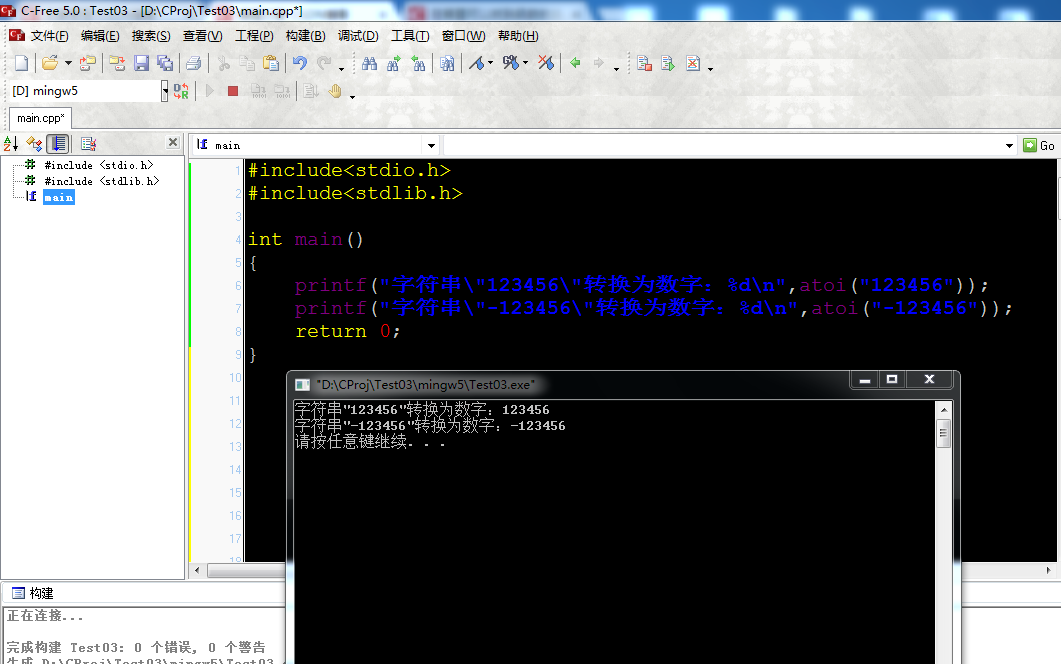2、自己简单实现

实现源码

#include<stdio.h>

int String2Int(char *str);//函数声明
int main()
{
printf("字符串\"123456\"转换为数字：%d\n",String2Int("123456"));
printf("字符串\"-123456\"转换为数字：%d\n",String2Int("-123456"));
return 0;
}

int String2Int(char *str)//字符串转数字
{
char flag = '+';//指示结果是否带符号
long res = 0;

if(*str=='-')//字符串带负号
{
++str;//指向下一个字符
flag = '-';//将标志设为负号
}
//逐个字符转换，并累加到结果res
while(*str>=48 && *str<57)//如果是数字才进行转换，数字0~9的ASCII码：48~57
{
res = 10*res+  *str++-48;//字符'0'的ASCII码为48,48-48=0刚好转化为数字0
}

if(flag == '-')//处理是负数的情况
{
res = -res;
}

return (int)res;
}


截图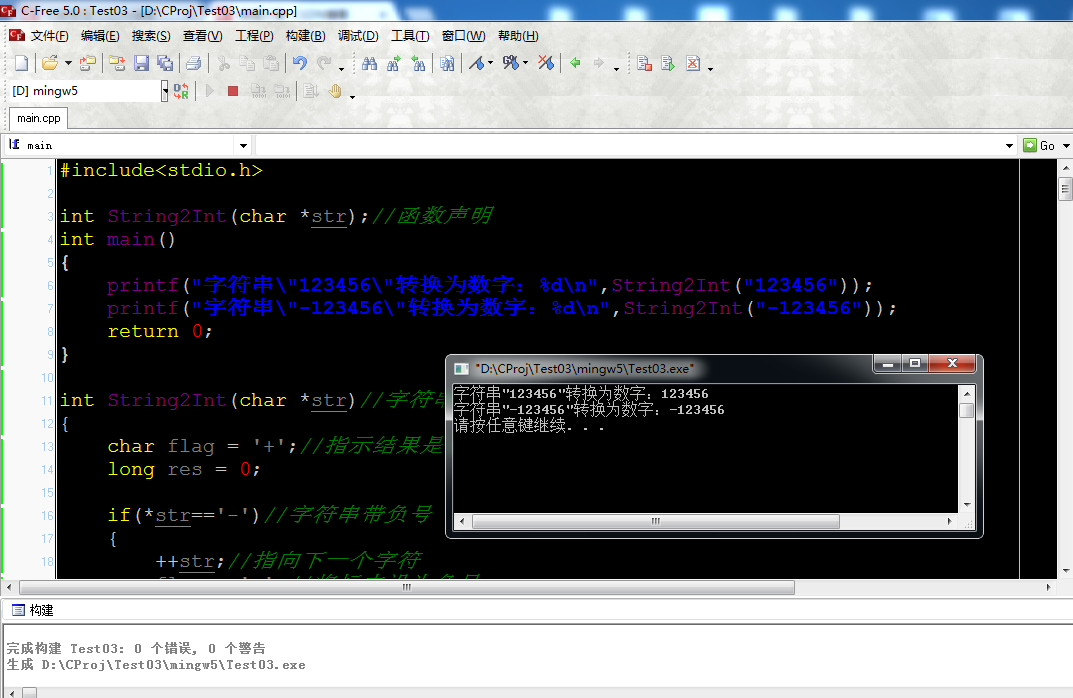四、利用sprintf()函数和sscanf()函数

整数转字符串

测试代码：

#include <stdio.h>

char* Int2String(int num,char *str);//函数声明
int main()
{
int number1 = 123456;
int number2 = -123456;
char string = {0};
Int2String(number1,string);
printf("数字：%d 转换后的字符串为：%s\n",number1,string);
Int2String(number2,string);
printf("数字：%d 转换后的字符串为：%s\n",number2,string);
return 0;
}

char* Int2String(int num,char *str)
{
sprintf(str, "%d", num);

return str;

}


运行结果：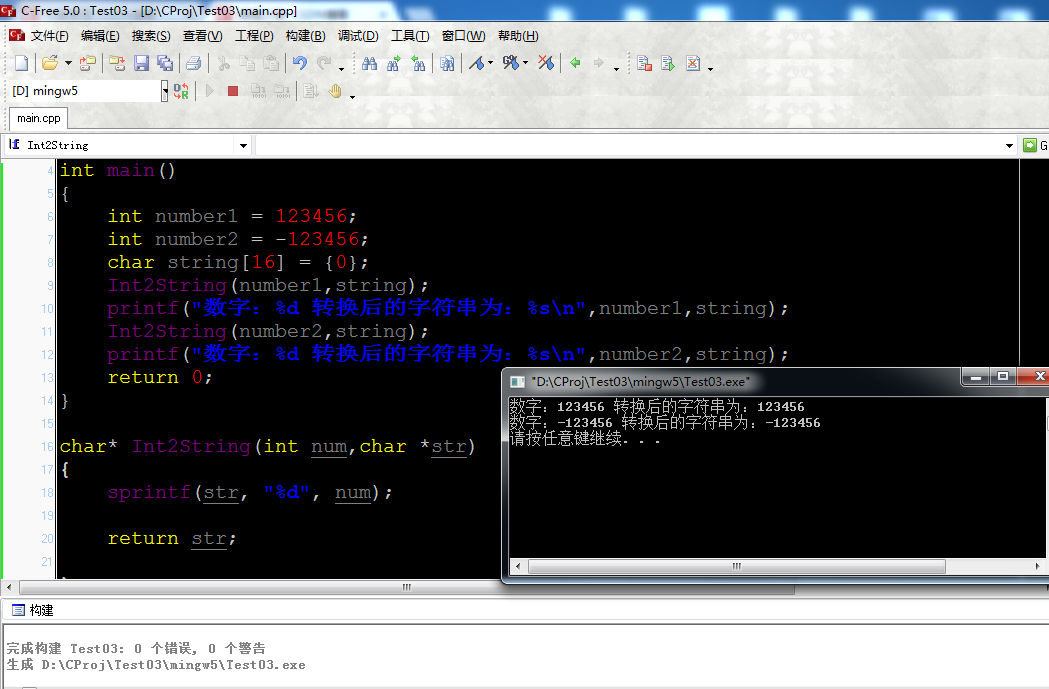字符串转整数

测试代码：

#include<stdio.h>

int String2Int(char *str);//函数声明
int main()
{
printf("字符串\"123456\"转换为数字：%d\n",String2Int("123456"));
printf("字符串\"-123456\"转换为数字：%d\n",String2Int("-123456"));
return 0;
}

int String2Int(char *str)//字符串转数字
{
char flag = '+';//指示结果是否带符号
long res = 0;

if(*str=='-')//字符串带负号
{
++str;//指向下一个字符
flag = '-';//将标志设为负号
}

sscanf(str, "%ld", &res);
if(flag == '-')
{
res = -res;
}
return (int)res;
}

运行结果：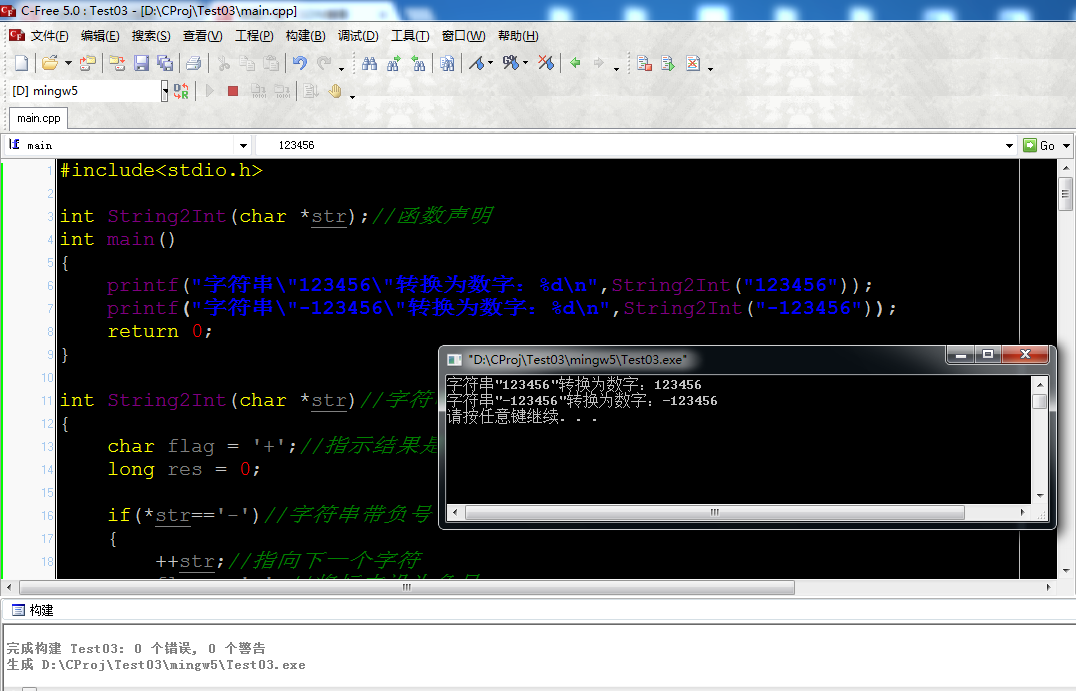五、附ASCII码表(部分)

ASCII值
控制字符
ASCII值
控制字符
ASCII值
控制字符
ASCII值
控制字符
0
NUT
32
(space)
64
@
96
、
1
SOH
33
!
65
A
97
a
2
STX
34
"
66
B
98
b
3
ETX
35
#
67
C
99
c
4
EOT
36
\$
68
D
100
d
5
ENQ
37
%
69
E
101
e
6
ACK
38
&
70
F
102
f
7
BEL
39
,
71
G
103
g
8
BS
40
(
72
H
104
h
9
HT
41
)
73
I
105
i
10
LF
42
*
74
J
106
j
11
VT
43
+
75
K
107
k
12
FF
44
,
76
L
108
l
13
CR
45
-
77
M
109
m
14
SO
46
.
78
N
110
n
15
SI
47
/
79
O
111
o
16
DLE
48
0
80
P
112
p
17
DCI
49
1
81
Q
113
q
18
DC2
50
2
82
R
114
r
19
DC3
51
3
83
S
115
s
20
DC4
52
4
84
T
116
t
21
NAK
53
5
85
U
117
u
22
SYN
54
6
86
V
118
v
23
TB
55
7
87
W
119
w
24
CAN
56
8
88
X
120
x
25
EM
57
9
89
Y
121
y
26
SUB
58
:
90
Z
122
z
27
ESC
59
;
91
[
123
{
28
FS
60
<
92
/
124
|
29
GS
61
=
93
]
125
}
30
RS
62
>
94
^
126

31
US
63
?
95
_
127
DEL

展开全文• C语言中的字符串都是以ASCII码的形式存放的。如数字‘1’在ASCII中存放的就是49.char i=9; int a; a=i; 上个例子中，你以为a得到的结果会是10，但是你运行一下发现其实这个时候i给a赋的值是 ‘59’。这时候想要...
    在C语言中的字符串都是以ASCII码的形式存放的。如数字‘1’在ASCII中存放的就是49.char i=9;
int a;
a=i;    上个例子中，你以为a得到的结果会是10，但是你运行一下发现其实这个时候i给a赋的值是 ‘59’。这时候想要得到你想要的数，也就是9方法有很多。    一、用int atoi（const char *）它会返回给你一个整数值。但是注意这个的头文件是在"#include<stdlib>"中的。               如 atoi('1234') ；//它会给你返回一个整形1234    二、因为整数型在ASCII码表中是线性排列的，及从48到57 对应着是 0到9；char i=9;
int a;
a=i-48;    我们在最后赋值时候加一个“-48”就可以得到单个整形数字。如果是在char 类型的数组中存放的，那么用把他们用十进制拼凑即可：char a[]='1234';
int b,i;
b=a-48;
for(i=1;i<4;i++)
b=b*10+a[i]-48;
printf("%d",b);

    上述写法也就可以自己写一个 atoi函数出来。
展开全文atoi函数
• 1.整形转字符串 A.无符号整形转字符串 void Int2Str(u8* str, u32 intnum) { u32 i, Div = 1000000000, j = 0, Status = 0; //32位无符号数最大是10位整数,所以Div=10 0000 0000 for (i = 0; i < 10; i++...
1.整形转字符串

A.无符号整形数转字符串

void Int2Str(u8* str, u32 intnum)
{
u32 i, Div = 1000000000, j = 0, Status = 0;
//32位无符号数最大是10位整数,所以Div=10 0000 0000
for (i = 0; i < 10; i++)
{
str[j++] = (intnum / Div) + '0';//取最高位 转化成字符

intnum = intnum % Div;//去掉最高位
Div /= 10;//还剩下10-i位要转换
if ((str[j-1] == '0') && (Status == 0))//忽略最高位的'0'
{
j = 0;
}
else
{
Status++;
}
}
}

u8 str[]={0};

Int2Str(str,2018);
printf("%s",str);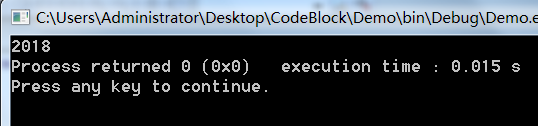B.有符号整形转字符串

/**
****************************************************************
* @brief int2str
*
* 功能：将整形(int)数据转换成字符串
*
* @param: n = 要转换的整形数据
* @param: str = 存放字符串的首地址
* @param: length = 转换后字符串的长度
*
****************************************************************/
void int2str(int n, char *str,unsigned char *length)
{
char buf = "";
int i = 0;
int len = 0;
// temp为n的绝对值
int temp = n<0?-n:n;
// 如果str为空，直接返回
if(str == NULL)
{
return ;
}
//把tmp的每一位的数存入buf
// 逆序存入，如1234 -> 在buf中为4321
//memset(CHAR,0,5);
while(temp)
{
buf[i++] = (temp%10) + '0';
temp = temp / 10;
}
//如果是负数多留一位放符串
len = n<0?++i:i;
*length = len;
str[i] = 0;
//反转字符串4321- 转成 -1234
while(1)
{
i--;
if(buf[len-i-1] == 0)
{
break;
}
str[i] = buf[len-i-1];
}
// 如果是符号，加回去
if(i==0)
{
str[i] = '-';//添加负号
}
}

2.字符串转整形

方法一（不推荐）：

#define IS_CAP_LETTER(c)    (((c) >= 'A') && ((c) <= 'F'))
#define IS_LC_LETTER(c)     (((c) >= 'a') && ((c) <= 'f'))
#define IS_09(c)            (((c) >= '0') && ((c) <= '9'))
#define ISVALIDHEX(c)       (IS_CAP_LETTER(c) || IS_LC_LETTER(c) || IS_09(c))
#define ISVALIDDEC(c)       IS_09(c)
#define CONVERTDEC(c)       (c - '0')

#define CONVERTHEX_ALPHA(c) (IS_CAP_LETTER(c) ? ((c) - 'A'+10) : ((c) - 'a'+10))
#define CONVERTHEX(c)       (IS_09(c) ? ((c) - '0') : CONVERTHEX_ALPHA(c))

u32 Str2Int(u8 *p_inputstr, u32 *p_intnum)
{
u32 i = 0, res = 0;
u32 val = 0;

if ((p_inputstr == '0') && ((p_inputstr == 'x') || (p_inputstr == 'X')))
{
i = 2;
while ( ( i < 11 ) && ( p_inputstr[i] != '\0' ) )
{
if (ISVALIDHEX(p_inputstr[i]))
{
val = (val << 4) + CONVERTHEX(p_inputstr[i]);
}
else
{
/* Return 0, Invalid input */
res = 0;
break;
}
i++;
}

/* valid result */
if (p_inputstr[i] == '\0')
{
*p_intnum = val;
res = 1;
}
}
else /* max 10-digit decimal input */
{
while ( ( i < 11 ) && ( res != 1 ) )
{
if (p_inputstr[i] == '\0')
{
*p_intnum = val;
/* return 1 */
res = 1;
}
else if (((p_inputstr[i] == 'k') || (p_inputstr[i] == 'K')) && (i > 0))
{
val = val << 10;
*p_intnum = val;
res = 1;
}
else if (((p_inputstr[i] == 'm') || (p_inputstr[i] == 'M')) && (i > 0))
{
val = val << 20;
*p_intnum = val;
res = 1;
}
else if (ISVALIDDEC(p_inputstr[i]))
{
val = val * 10 + CONVERTDEC(p_inputstr[i]);
}
else
{
/* return 0, Invalid input */
res = 0;
break;
}
i++;
}
}
return res;
}

u8 strs[]="123456";

Str2Int(strs,&Num);
printf("%d\r\n",Num);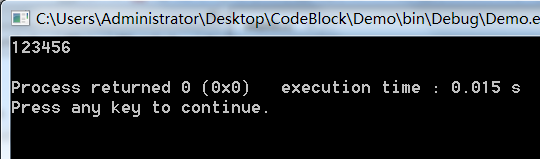方法二（转载另一位网友的）：

这里给出原文地址：https://blog.csdn.net/second60/article/details/80503335

int str2int1( char* str)
{
int temp = 0;
const char* p = str;
if(str == NULL) return 0;
if(*str == '-' || *str == '+')
{
str ++;
}
while( *str != 0)
{
if( *str < '0' || *str > '9')
{
break;
}
temp = temp*10 +(*str -'0');
str ++;
}
if(*p == '-')
{
temp = -temp;
}
return temp;
}

`
展开全文• C语言中的字符串 C语言中的字符串 从概念上将，C语言中没有字符串数据类型 在C语言中使用字符数组来模拟字符串 C语言字符串是以’\0’结束的字符数组 C语言中的字符串可以分配在栈空间、堆空间、只读存储区 4-1.c#...
• 一、用一维字符数组存放字符串1、C语言字符串的约定字符串是借助于字符型一维数组来存放的，并规定以字符‘\0’作为字符串的结束标志，’\0’作为标志占用存储空间，但不计入串的实际常量2、C语言中表示字符串常量...
• 每个枚举常量对应一个整形...#会将后面的内容作为字符串返回如果想让#后面的内容作为宏使用，需要使用两层宏展开，且使用宏使用的宏里面的每个字符见到的字符都会作为返回字符串的一部分。如printf("10->%s\r\n...
• 格式字符串的形式为： % [输出最小宽度] [.精度] [长度] 类型例如，%d格式符表示 用十进制整形格式输出。%f表示用实型格式输出，%5.2f 格式表示输出宽度为5(包括小数点)，并包含2位小数。常用的输出格式及含义如下:...
• C语言中字符和字符串的定义，及其相关的函数，包括复制，比较，转换成整形数等
• #include double atof(const char *nptr);...将字符串转换成整形数long atol(const char *nptr);将字符串转换成长整型数double strtod(const char *nptr, char **endptr);将字符串转换成双精度浮点数long int str...
• r习题，将16进制数(可选0x或0X前缀)组成的字符串转换成等价的整型,写了一个：/* htoi.c* transform hex to decimal** compile with my_getline.c*/#include#define MAXLEN 20int my_getline(char s[], int lim);...
• //将字符串转换为整型值。 int atoi(char s[]) {  int i, n, sign = 1;  for (i = 0; myIsspace(s[i]); i++);//跳过空白符  sign = (s[i] == '-') ? -1 : 1;  if (s[i] == '+' || s[i] == '-')//跳过符号 ...
• C++中如何将整型变量转换为字符串int i=1;...C语言中将整形变量转换成字符串的函数是什么?int iValue;//整型数 char sz;//字符串 sprintf(sz, "%d", iValue);//这句需要头文件#include /*或者*/ itoa(iValue, sz...
• ## C语言数组之字符串

千次阅读 多人点赞 2018-11-20 01:55:35
最近各大工作室都发布了一些与字符串有关的题目，同学们在写有关字符串的题目时候会感到吃力，或是无从下手，没有思路，或是一些基本的知识不明白。这里我就讲解下与字符串有关的基本知识 字符串，它是由多个字符...
• C语言提供了几个标准库函数，可以将任意类型(整型、长整型、浮点型等)的数字转换为字符串。 1.int/float to string/array: C语言提供了几个标准库函数，可以将任意类型(整型、长整型、浮点型等)的数字转换为...
• 整型转字符 int n; scanf("%d",&amp;n); char c; c = (char)n; printf("%c\n",c); 输入65 输出A，以为A的ascii码为65 字符转整型 不能强制类型转换 //直接相减 char c; ...
• C语言回炉之字符串和格式化输入输出char[] 和 null字符串 和 字符defineconst明示常量printf转换说明符printf转换修饰符printf转换标记scanf的转换说明符scanf的转换修饰符printf 和scanf的*修饰符printf() 的*修饰...c printf scanf
• atoi这个函数的实现要考虑正负还有字母等情况下面是字符串转换成整型数#include #include int my_atio(char *String);int main(){char String;int num;printf("please input a string:\n");scanf("%s",String);...
• 问题： 对于字符串char* = " abcd efg h"; 要求输出" h efg abcd ";...将字符串第一个出现空格位置的索引记录下来，并且放到一个整形数组里，例如上面的出现空格的位置分别是0 5 11 那么记录数组int b[j]; b=0 ...
• 比如这样一个问题：输入一个正整数，将它转为正整数。 可用sprintf、snprint来实现。代码如下： #include #include int a=0; char buffer; int main() { do { printf("input an ... } while(a)语言 buffer c input
• 一维数组，数组是C语言中为了 ，专门定义大量的同种变量的一种特殊方式。现在设置一个整形的数组z[n];数组的定义，和一些的单个变量一样都是分类型的。比如整形变量的数组，里面只能存放着整形的变量。所以在定义的...数组的复制
• C语言提供了几个标准库函数，可以将任意类型(整型、长整型、浮点型等)的数字转换为字符串。以下是用itoa()函数将整数 换为字符串的一个例子：  # include  # include    void main (void)  {  int num ...printf windows string
• c语言字符串中的小写字母转换成大写字母描述给定一个字符串，将其中所有的小写字母转换成大写字母。输入输入一行，包含一个字符串(长度不超过100，可能包含空格)。输出输出转换后的字符串。样例输入helloworld123...
• C语言字符串的定义字符串是一个或多个字符的序列。"rumenz.com"双引号不是字符串的一部分, 双引号仅告知编译器它括起来的是字符串，正如单引号用户标识单个字符。C语言字符串和字符的关系C语言没有专门的字符串类型...
• 文章目录字符函数和字符串函数字符串基础知识函数介绍长度不受限制的字符串函数strlen(字符串长度)strcpy(字符串拷贝)strcat(连接字符串)strcmp（字符串比较）长度受限制的字符串函数介绍strncpystrncatstrncmp字符...
• strcmp函数实际上是对字符的ASCII码进行比较 , 其中str1和str2可以是字符串常量或者字符串变量，返回值为整形,所以区分大小写。① str1&lt;str2，返回负值或者-1； ② str1=str2，返回0...
• ## [C语言]数字字符串转换成整型

万次阅读 多人点赞 2018-09-24 16:24:54
数字字符串转换成整型 我们经常会碰到将数字字符串转换成整型的问题，此篇文章给出一种常见的解决方案 知识补充： ‘1’ - ‘0’ = 1 str2int.c #include&amp;lt;stdio.h&amp;gt; #include&amp...
• 一、字符串 3、字符串特点 a) 字符串占位符是%s b)字符串占用内存是连续的，一个字符挨着一个字符 一旦碰到‘\0’则表示字符串的结束 字符串有点像数组，内存也是连续的 例如 printf(“%s\n”,”abcd\0def”);//...指针 算法...

# c语言整形转字符串c语言 订阅